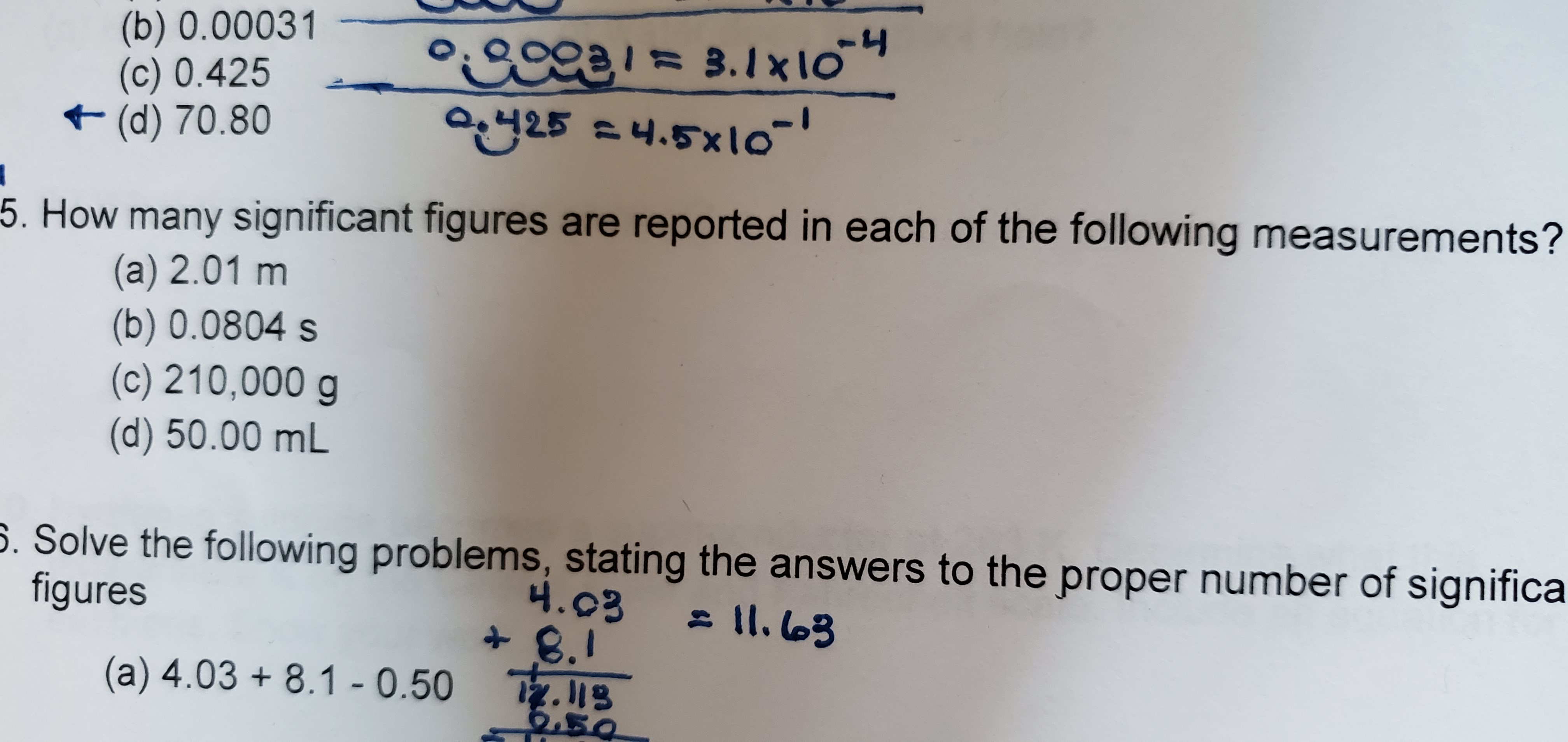# (b) 0.00031(c) 0.425(d) 70.80°COca= 3.1x lo254.5105. How many significant figures are reported in each of the following measurements?(a) 2.01 m(b) 0.0804 s(c) 210,000 g(d) 50.00 mL5. Solve the following problems, stating the answers to the proper number of significafigures4.03- lI. l03+8.1B.I(a) 4.03 8.1 - 0.50Tz.118

Question
8 viewshelp_outlineImage Transcriptionclose(b) 0.00031 (c) 0.425 (d) 70.80 °COca= 3.1x lo 254.510 5. How many significant figures are reported in each of the following measurements? (a) 2.01 m (b) 0.0804 s (c) 210,000 g (d) 50.00 mL 5. Solve the following problems, stating the answers to the proper number of significa figures 4.03 - lI. l03 +8.1 B.I (a) 4.03 8.1 - 0.50 Tz.118 fullscreen
check_circle

Step 1

(a)

Significant figure is a non-zero number and zero placed in between non-zero numbers is significant figure.

Number of significant figures is 3.

Step 2

(b)

Zeros before the non-zero number are not significant number and zero placed in between non-zero numbers is significant figure.

Number of significant figures is 3.

Step 3

(c)

Zeros after the non-zero number are significant in case of that number c...

### Want to see the full answer?

See Solution

#### Want to see this answer and more?

Solutions are written by subject experts who are available 24/7. Questions are typically answered within 1 hour.*

See Solution
*Response times may vary by subject and question.
Tagged in

### General Chemistry